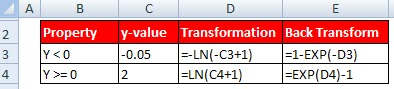# Regression : Transform Negative Values

In linear regression, box-cox transformation is widely used to transform target variable so that linearity and normality assumptions can be met. But box-cox transformation can be used only for strictly positive target values. If you have negative values in your target (dependent) variable, the box-cox and log transformation cannot be used.

How to handle negative data values

1. Cube Root (Power- 1/3)
Cube root can be used to transform negative, zero and positive data values. The best part about this transformation is it is very easy to perform 'back transformation' of this form to get back real values.

Back Transformation : Cube of the transformed value

2. Yeo-Johnson Power Transformations

It is an extension of Box cox transformation. It allows transformation of negative values.

R Code :
require(car)
lambda.fm1 <- boxcox(mydata\$y ~ mydata\$x1 + mydata\$x2), family="yjPower")
lambda.max <- lambda.fm1\$x[which.max(lambda.fm1\$y)]
It can be easily implemented manually. Look at the property shown below :
For Y  <  0 ===> - log( -y + 1)
For Y >= 0 ===>   log( y + 1)Yeo-Johnson Power Transformation
LN : Natural Log (base e)

With both negative and positive values, the transformation is a mixture of these
two, so different powers are used for positive and negative values. In this latter case,
interpretation of the transformation parameter is difficult, as it has a different meaning
for y<0 and y>=0.

= log(1+Y-min(Y))
Note : Both log to base e and log to base 10 can be used.

Back Transformation :  = exp(transformed value) -1+ min(Y)Share
Related PostsDeepanshu founded ListenData with a simple objective - Make analytics easy to understand and follow. He has over 10 years of experience in data science. During his tenure, he has worked with global clients in various domains like Banking, Insurance, Private Equity, Telecom and Human Resource.

2 Responses to "Regression : Transform Negative Values"
1.Isn't the cube root of a negative number another negative number too?

1.Yeah, the point is to try and squish the numbers together in reversible ways to make them "look" as normal as possible. The center of that normal distribution can be wherever on the number line.

Next → ← Prev